Next: Example 10.2: Energy density Up: Inductance Previous: Worked Examples

## Example 10.1: Mutual induction

Question: Suppose that two insulated wires are wound onto a common cylindrical former of length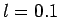m and cross-sectional area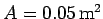. The number of turns in the first wire is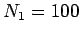, and the number of turns in the second wire is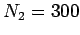. What is the mutual inductance of the two wires? If the current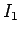flowing in the first wire increases uniformly from 0 to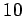A in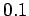s, what emf is generated in the second wire? Does this emf act to drive a current in the second wire which circulates in the same sense as the current in the first wire, or the opposite sense?

Answer: From Eq. (240), the mutual inductance of the two wires is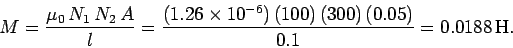From Eq. (236), the emf generated around the second loop by the changing current in the first loop is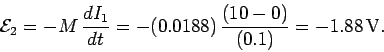The minus sign indicates that this emf acts so as to drive a current in the second wire which circulates in the opposite sense to the current flowing in the first wire, in accordance with Lenz's law. If the current in the first wire were decreased, instead of increased, then the emf in the second wire would act to drive a current which circulates in the same sense as the former current.Next: Example 10.2: Energy density Up: Inductance Previous: Worked Examples
Richard Fitzpatrick 2007-07-14